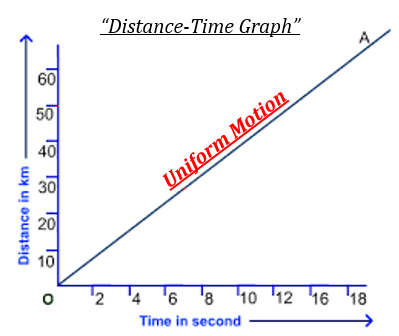# Define Uniform Motion.

Uniform Motion- This type of motion is defined as, if a body travels in a straight line and its velocity remains constant along that line as it covers equal distances in equal intervals of time, no matter how small these time intervals may be.

If we draw a distance-time graph for uniform motion then it will be a straight line.Updated on: 10-Oct-2022

145 Views